# Laminar flow

TOO SHORTThis article was checked by pedagogue, but more than year ago. Signature:  Carmeljcaruana (talk)
Article to be checked

Laminar flow is one of three types of flow in fluid dynamics (laminar, turbulent and transitional) which is characterised by the layers of the fluid running in straight lines parallel to one another and the sides of the pipe, e.g. like a pack of cards. There is no intermixing between layers and the flow is seen as very regular.Laminar flow, highest velocity in the centre, lowest near the sides of the pipe.

## Determining type of flow

Whether the flow of a fluid is laminar, turbulent or transitional can be calculated by finding its Reynolds number, Re, which is also a dimensionless number in that it has no units.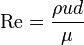$\mathrm{Re} = {{\rho {ud} } \over {\mu}}$

where:

•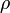$\rho$ = density of fluid (kg/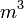$m^3$)
•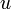${u}$ = mean velocity of fluid (m/s)
•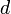${d}$ = diameter of pipe (m)
•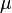$\mu$ = viscosity of fluid (kg/ms)

Laminar flow generally occurs when the Reynolds number is less than 2000. Turbulent flow occurs at an Re above 4000 and transitional flow occurs between 2000 and 4000. As can be seen from the formula, as the density and velocity of the fluid and the diameter of the pipe increase, so does the tendency towards turbulent flow, while increasing the viscosity of the fluid increases the tendency towards laminar flow.

The equation for Reynolds number can also be seen as the ratio between inertial and viscous forces in the fluid. The more the ratio is in favour of inertial forces, the higher Reynolds number and vice versa.

## Clinical significance of laminar flow

Blood flow through vessels is generally laminar. Because of the lack of interaction between concentric layers of blood, there is minimal energy loss. However when arteries become diseased and plaque builds up on the lumen (atherosclerosis) or when heart valves thicken narrowing the diameter of passage, flow becomes more turbulent and more energy is lost from interactions between layers of fluid. This can result in an abnormal heart murmur which can be heard through a stethoscope and can be a sign of heart valve disease.